|

# 上海这个地方,国庆宁愿不去旅游也要抢着去!

告诉大家一个好消息：再上三天班就要放!假!啦!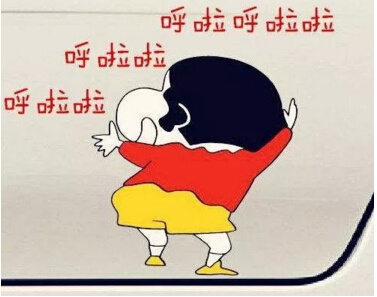作为一年中屈指可数的长假，国庆中秋一到，宅了一年的广大人民群众就义无反顾地投入到旅游的汪洋大海中，妥妥地开启“人从众”模式。各种平日里美不胜收的风景，此时却变成“一眼望去皆人头，高矮胖瘦各不同”的人海，让人郁闷不已。

国庆想去嗨，又不想人挤人，怎么办?教你避免“人从众”假期模式的正确姿势：

留 在 上 海

其实，上海国庆假期有个神秘好去处，吃喝玩乐样样有，还不用跑远去挤，今晚就给大家提前透露下，提前收藏好噢!

神秘好去处：装修嘉年华，国庆不打烊!(同期举办2017中国华夏家博会)

坐标点：世博展览馆

时间轴：10月1-3日

非看不可的理由： 最IN潮流家居品牌，打造吃喝玩乐超爽体验!

独家体验指南：10大最IN品牌联合推出不同于卖场、团购会纯卖货的特色互动体验，把对家居生活的热爱植入观众心中，感受家居生活所蕴藏的乐趣。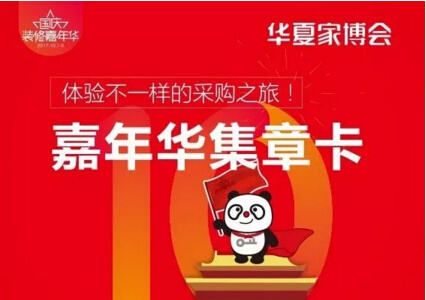完成任务奖励：观众现场可免费领取[嘉年华集章卡]，到各展厅完成体验便可收带“装”、“修”、“嘉”、“年”、“华”、“国”、“庆”、“不”、“打”、“烊”任意字样盖章一枚;集满相应字章，可获超多超丰富礼品奖励!

嘉年华第一站：方太厨电

特色体验：DIY果汁制作

位置分布：世博展览馆中厅北面

互动内容：活动期间，至方太展区参与果蔬清洗DIY自制果汁游戏，即可集章一枚。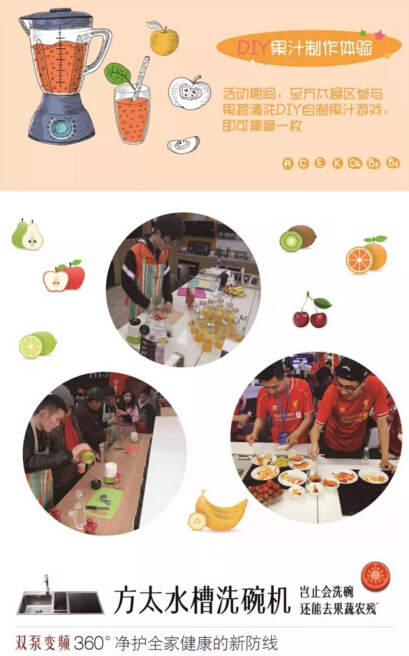嘉年华第二站：老板电器

特色体验：厨房美丽之旅

位置分布：世博展览馆中厅北面

互动内容：嘴唇彩绘(体验酱嘴唇涂鸦)、一键煎蛋(进行一键煎蛋互动)、皮肤检测(皮肤专家现场检测)、性感美食制作(多彩少女马卡龙)，以上4个项目，体验任意一项即可集章一枚。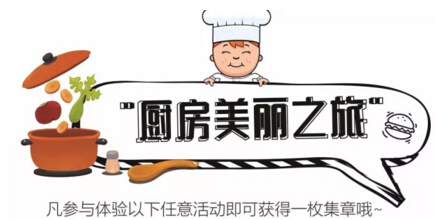嘉年华第三站：西门子电器

特色体验：咖啡手工制

位置分布：世博展览馆H1馆TA46

互动内容：一键花式咖啡制作，无论是香浓的拿铁，还是奶泡绵密的卡布奇诺，只需轻轻一按，花式咖啡顷刻呈现，成功制作后可集章一枚。嘉年华第四站：博世电器

特色体验：披萨烘焙赏

位置分布：世博展览馆H1馆TA81

互动内容：色拉米披萨。1、提供准备食材(灯笼红椒、灯笼黄椒、白洋葱、番茄、番茄酱、马苏里拉奶酪、自制饼底)。2、用博世厨师机调制五档，开启搅拌功能将面粉、水、酵母、糖、盐进行均匀搅拌，并赶制成饼皮，放入烤箱中烤熟备用。3、将灯笼红、黄椒、白洋葱用手持式搅拌棒切丝，番茄切粒备用。4、接着在饼皮上面均匀涂抹番茄酱、撒上备料、芝士，最后铺上色拉米肠。5、博世烤箱提前调制，顶部/底部加热215度，烘烤7分钟即成。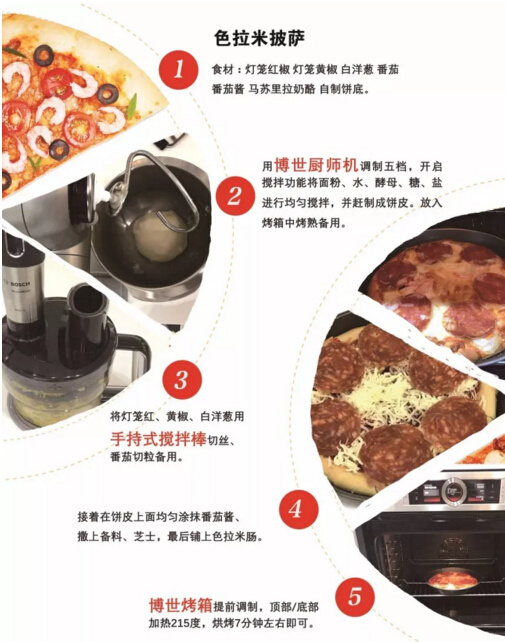嘉年华第五站：冠珠瓷砖

特色体验：瓷砖拼拼乐

位置分布：世博展览馆H1馆TA05

互动内容：冠珠提供印制有Angelababy和嘉年华主题的定制瓷砖，按图所示把16块不同图案的零散图案瓷砖按照顺序拼齐，即可集章一枚。嘉年华第六站：马可波罗瓷砖

特色体验：幸运射飞镖

位置分布：世博展览馆H1馆TA23

互动内容：参与单人幸运射飞镖游戏，射中什么，就送什么!一等奖(投中9环)：马可波罗瓷砖青花瓷茶具一套;二等奖(投中7/8环)：马可波罗瓷砖高级双人雨伞一把;三等奖(投中4/6环)：精美蘑菇杯一个;四等奖(投中1/2/3环)：金属手机指环支架一个。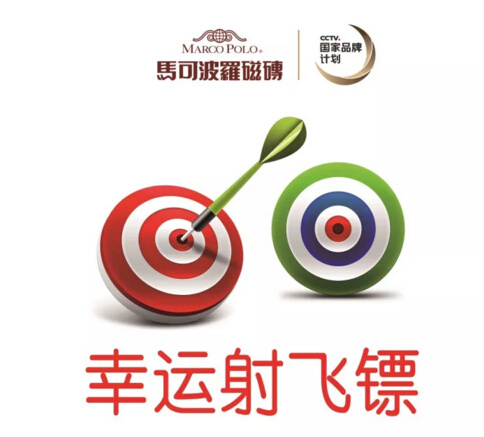嘉年华第七站：立升净水

特色体验：净水科普实验

位置分布：世博展览馆H1馆TA68-1

互动内容：净水技术太抽象?净化效果看不见?来吧!科普小实验，亲身体验，解开心中的疑惑。PS：有工作人员辅导噢!NO1：膜丝原理实验：超滤膜过滤;NO2：净水器排污实验：净水排污原理;NO3：水质试剂实验：水质化学小实验;NO4：单兵制水实验：户外便携式净水制取。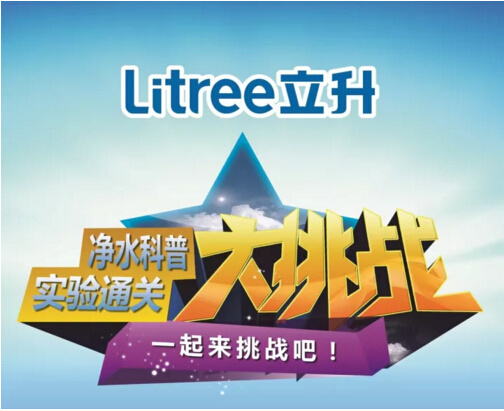嘉年华第八站：德国都芳漆

特色体验：灵动的金鱼

位置分布：世博展览馆H1馆TA70-1

互动内容：寻找德国都芳漆吉祥物刺猬+用都芳漆喂养的金鱼，拍照分享到朋友圈即送刺猬公仔一个。嘉年华第九站：壹居儿童家居

特色体验：娱乐大作战

位置分布：世博展览馆H2馆TB53-1

互动内容：1、滑滑梯：小型的儿童滑滑梯;2、儿童沙画：打造美好亲子时光;3、沙土玩具：让孩子自由玩乐。体验上述任意一项即可集章一枚。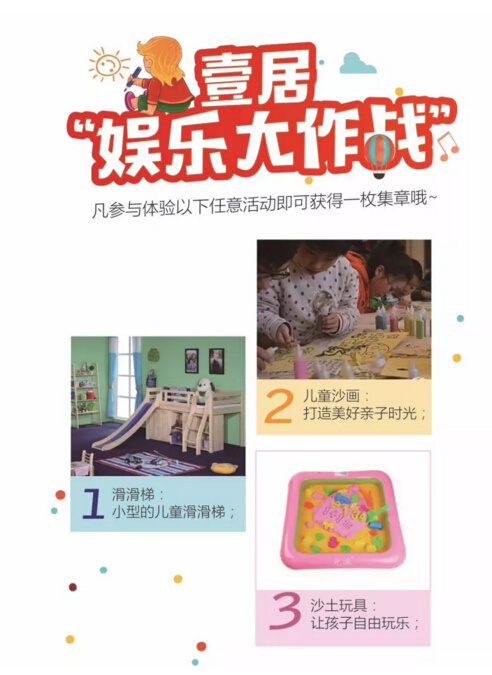嘉年华第十站：北疆硅藻泥

特色体验：手工捏泥人

位置分布：世博展览馆H2馆TB74

互动内容：凡进展位并参与“重拾儿梦——玩泥巴”的观众，均可集章一枚。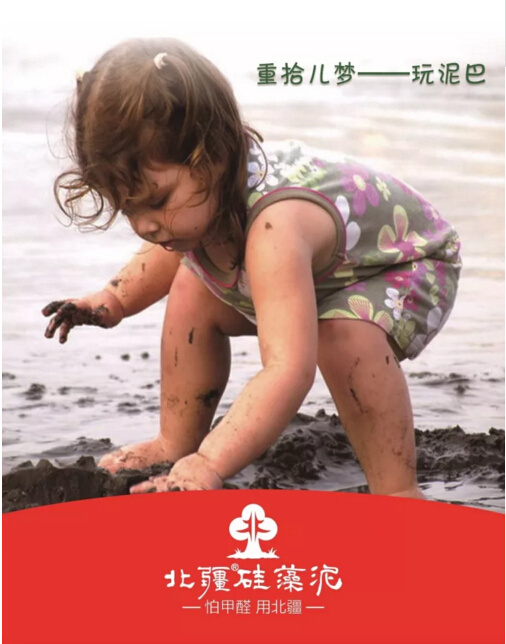最后提醒去体验的观众，一定要提前预定免费门票(现场购票10元/位)

`声明：本文由入驻焦点开放平台的作者撰写，除焦点官方账号外，观点仅代表作者本人，不代表焦点立场错误信息举报电话： 400-099-0099，邮箱：jubao@vip.sohu.com，或点此进行意见反馈，或点此进行举报投诉。`A B C D E F G H J K L M N P Q R S T W X Y Z
A - B - C - D - E
• A
• 鞍山
• 安庆
• 安阳
• 安顺
• 安康
• 澳门
• B
• 北京
• 保定
• 包头
• 巴彦淖尔
• 本溪
• 蚌埠
• 亳州
• 滨州
• 北海
• 百色
• 巴中
• 毕节
• 保山
• 宝鸡
• 白银
• 巴州
• C
• 承德
• 沧州
• 长治
• 赤峰
• 朝阳
• 长春
• 常州
• 滁州
• 池州
• 长沙
• 常德
• 郴州
• 潮州
• 崇左
• 重庆
• 成都
• 楚雄
• 昌都
• 慈溪
• 常熟
• D
• 大同
• 大连
• 丹东
• 大庆
• 东营
• 德州
• 东莞
• 德阳
• 达州
• 大理
• 德宏
• 定西
• 儋州
• 东平
• E
• 鄂尔多斯
• 鄂州
• 恩施
F - G - H - I - J
• F
• 抚顺
• 阜新
• 阜阳
• 福州
• 抚州
• 佛山
• 防城港
• G
• 赣州
• 广州
• 桂林
• 贵港
• 广元
• 广安
• 贵阳
• 固原
• H
• 邯郸
• 衡水
• 呼和浩特
• 呼伦贝尔
• 葫芦岛
• 哈尔滨
• 黑河
• 淮安
• 杭州
• 湖州
• 合肥
• 淮南
• 淮北
• 黄山
• 菏泽
• 鹤壁
• 黄石
• 黄冈
• 衡阳
• 怀化
• 惠州
• 河源
• 贺州
• 河池
• 海口
• 红河
• 汉中
• 海东
• I
• J
• 晋中
• 锦州
• 吉林
• 鸡西
• 佳木斯
• 嘉兴
• 金华
• 景德镇
• 九江
• 吉安
• 济南
• 济宁
• 焦作
• 荆门
• 荆州
• 江门
• 揭阳
• 金昌
• 酒泉
• 嘉峪关
K - L - M - N - P
• K
• 开封
• 昆明
• 昆山
• L
• 廊坊
• 临汾
• 辽阳
• 连云港
• 丽水
• 六安
• 龙岩
• 莱芜
• 临沂
• 聊城
• 洛阳
• 漯河
• 娄底
• 柳州
• 来宾
• 泸州
• 乐山
• 六盘水
• 丽江
• 临沧
• 拉萨
• 林芝
• 兰州
• 陇南
• M
• 牡丹江
• 马鞍山
• 茂名
• 梅州
• 绵阳
• 眉山
• N
• 南京
• 南通
• 宁波
• 南平
• 宁德
• 南昌
• 南阳
• 南宁
• 内江
• 南充
• P
• 盘锦
• 莆田
• 平顶山
• 濮阳
• 攀枝花
• 普洱
• 平凉
Q - R - S - T - W
• Q
• 秦皇岛
• 齐齐哈尔
• 衢州
• 泉州
• 青岛
• 清远
• 钦州
• 黔南
• 曲靖
• 庆阳
• R
• 日照
• 日喀则
• S
• 石家庄
• 沈阳
• 双鸭山
• 绥化
• 上海
• 苏州
• 宿迁
• 绍兴
• 宿州
• 三明
• 上饶
• 三门峡
• 商丘
• 十堰
• 随州
• 邵阳
• 韶关
• 深圳
• 汕头
• 汕尾
• 三亚
• 三沙
• 遂宁
• 山南
• 商洛
• 石嘴山
• T
• 天津
• 唐山
• 太原
• 通辽
• 铁岭
• 泰州
• 台州
• 铜陵
• 泰安
• 铜仁
• 铜川
• 天水
• 天门
• W
• 乌海
• 乌兰察布
• 无锡
• 温州
• 芜湖
• 潍坊
• 威海
• 武汉
• 梧州
• 渭南
• 武威
• 吴忠
• 乌鲁木齐
X - Y - Z
• X
• 邢台
• 徐州
• 宣城
• 厦门
• 新乡
• 许昌
• 信阳
• 襄阳
• 孝感
• 咸宁
• 湘潭
• 湘西
• 西双版纳
• 西安
• 咸阳
• 西宁
• 仙桃
• 西昌
• Y
• 运城
• 营口
• 盐城
• 扬州
• 鹰潭
• 宜春
• 烟台
• 宜昌
• 岳阳
• 益阳
• 永州
• 阳江
• 云浮
• 玉林
• 宜宾
• 雅安
• 玉溪
• 延安
• 榆林
• 银川
• Z
• 张家口
• 镇江
• 舟山
• 漳州
• 淄博
• 枣庄
• 郑州
• 周口
• 驻马店
• 株洲
• 张家界
• 珠海
• 湛江
• 肇庆
• 中山
• 自贡
• 资阳
• 遵义
• 昭通
• 张掖
• 中卫

1室1厅1厨1卫1阳台

1
2
3
4
5

0
1
2

1

1

0
1
2
3报名成功，资料已提交审核A B C D E F G H J K L M N P Q R S T W X Y Z
A - B - C - D - E
• A
• 鞍山
• 安庆
• 安阳
• 安顺
• 安康
• 澳门
• B
• 北京
• 保定
• 包头
• 巴彦淖尔
• 本溪
• 蚌埠
• 亳州
• 滨州
• 北海
• 百色
• 巴中
• 毕节
• 保山
• 宝鸡
• 白银
• 巴州
• C
• 承德
• 沧州
• 长治
• 赤峰
• 朝阳
• 长春
• 常州
• 滁州
• 池州
• 长沙
• 常德
• 郴州
• 潮州
• 崇左
• 重庆
• 成都
• 楚雄
• 昌都
• 慈溪
• 常熟
• D
• 大同
• 大连
• 丹东
• 大庆
• 东营
• 德州
• 东莞
• 德阳
• 达州
• 大理
• 德宏
• 定西
• 儋州
• 东平
• E
• 鄂尔多斯
• 鄂州
• 恩施
F - G - H - I - J
• F
• 抚顺
• 阜新
• 阜阳
• 福州
• 抚州
• 佛山
• 防城港
• G
• 赣州
• 广州
• 桂林
• 贵港
• 广元
• 广安
• 贵阳
• 固原
• H
• 邯郸
• 衡水
• 呼和浩特
• 呼伦贝尔
• 葫芦岛
• 哈尔滨
• 黑河
• 淮安
• 杭州
• 湖州
• 合肥
• 淮南
• 淮北
• 黄山
• 菏泽
• 鹤壁
• 黄石
• 黄冈
• 衡阳
• 怀化
• 惠州
• 河源
• 贺州
• 河池
• 海口
• 红河
• 汉中
• 海东
• I
• J
• 晋中
• 锦州
• 吉林
• 鸡西
• 佳木斯
• 嘉兴
• 金华
• 景德镇
• 九江
• 吉安
• 济南
• 济宁
• 焦作
• 荆门
• 荆州
• 江门
• 揭阳
• 金昌
• 酒泉
• 嘉峪关
K - L - M - N - P
• K
• 开封
• 昆明
• 昆山
• L
• 廊坊
• 临汾
• 辽阳
• 连云港
• 丽水
• 六安
• 龙岩
• 莱芜
• 临沂
• 聊城
• 洛阳
• 漯河
• 娄底
• 柳州
• 来宾
• 泸州
• 乐山
• 六盘水
• 丽江
• 临沧
• 拉萨
• 林芝
• 兰州
• 陇南
• M
• 牡丹江
• 马鞍山
• 茂名
• 梅州
• 绵阳
• 眉山
• N
• 南京
• 南通
• 宁波
• 南平
• 宁德
• 南昌
• 南阳
• 南宁
• 内江
• 南充
• P
• 盘锦
• 莆田
• 平顶山
• 濮阳
• 攀枝花
• 普洱
• 平凉
Q - R - S - T - W
• Q
• 秦皇岛
• 齐齐哈尔
• 衢州
• 泉州
• 青岛
• 清远
• 钦州
• 黔南
• 曲靖
• 庆阳
• R
• 日照
• 日喀则
• S
• 石家庄
• 沈阳
• 双鸭山
• 绥化
• 上海
• 苏州
• 宿迁
• 绍兴
• 宿州
• 三明
• 上饶
• 三门峡
• 商丘
• 十堰
• 随州
• 邵阳
• 韶关
• 深圳
• 汕头
• 汕尾
• 三亚
• 三沙
• 遂宁
• 山南
• 商洛
• 石嘴山
• T
• 天津
• 唐山
• 太原
• 通辽
• 铁岭
• 泰州
• 台州
• 铜陵
• 泰安
• 铜仁
• 铜川
• 天水
• 天门
• W
• 乌海
• 乌兰察布
• 无锡
• 温州
• 芜湖
• 潍坊
• 威海
• 武汉
• 梧州
• 渭南
• 武威
• 吴忠
• 乌鲁木齐
X - Y - Z
• X
• 邢台
• 徐州
• 宣城
• 厦门
• 新乡
• 许昌
• 信阳
• 襄阳
• 孝感
• 咸宁
• 湘潭
• 湘西
• 西双版纳
• 西安
• 咸阳
• 西宁
• 仙桃
• 西昌
• Y
• 运城
• 营口
• 盐城
• 扬州
• 鹰潭
• 宜春
• 烟台
• 宜昌
• 岳阳
• 益阳
• 永州
• 阳江
• 云浮
• 玉林
• 宜宾
• 雅安
• 玉溪
• 延安
• 榆林
• 银川
• Z
• 张家口
• 镇江
• 舟山
• 漳州
• 淄博
• 枣庄
• 郑州
• 周口
• 驻马店
• 株洲
• 张家界
• 珠海
• 湛江
• 肇庆
• 中山
• 自贡
• 资阳
• 遵义
• 昭通
• 张掖
• 中卫• 手机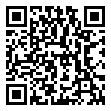• 分享
• 设计
免费设计
• 计算器
装修计算器
• 入驻
合作入驻
• 联系
联系我们
• 置顶
返回顶部